802.11az Positioning Using Super-Resolution Time of Arrival Estimation

This example shows how to estimate the position of a station (STA) in a multipath environment by using a time-of-arrival-based (ToA-based) positioning algorithm defined in the IEEE® 802.11az™ Wi-Fi™ standard. The example estimates the ToA by using a multiple signal classification (MUSIC) super-resolution approach, then estimates the two-dimensional position of a STA by using trilateration. The example then evaluates and compares the performance of the positioning algorithm at multiple signal-to-noise ratio (SNR) points.

Introduction

The 802.11az standard , commonly referred to as next generation positioning (NGP), enables a STA to identify its position relative to multiple access points (APs). This standard supports two high-efficiency (HE) ranging physical layer (PHY) protocol data unit (PPDU) formats:

• HE ranging null data packet (NDP)

• HE trigger-based (TB) ranging NDP

The HE ranging NDP and HE TB ranging NDP are the respective analogues of the HE sounding NDP and HE TB feedback NDP PPDU formats, as defined in the 802.11ax™ standard. For more information on these HE PPDU formats, see .

The HE ranging NDP supports the positioning of one or more users with an optional secure HE long training field (HE-LTF) sequence. The single-user HE ranging waveform contains HE-LTF symbols for a single user, which also support an optional secure HE-LTF sequence. The multi-user HE ranging waveform permits only secure HE-LTF symbols for multiple users. To improve position estimation accuracy, single-user and multi-user waveforms can contain multiple repetitions of the HE-LTF symbols. To parameterize and generate HE ranging NDPs, see the 802.11az Waveform Generation example.

This example simulates an 802.11az network consisting of a STA and multiple APs. To estimate the position of a STA, the network requires a minimum of three APs. The example simulates a ranging measurement exchange for each STA-AP pair, then trilaterates the position of the STA by using these measurements. The example repeats this simulation for multiple iterations and SNR points. This diagram shows the positioning process in a network with one STA and three APs.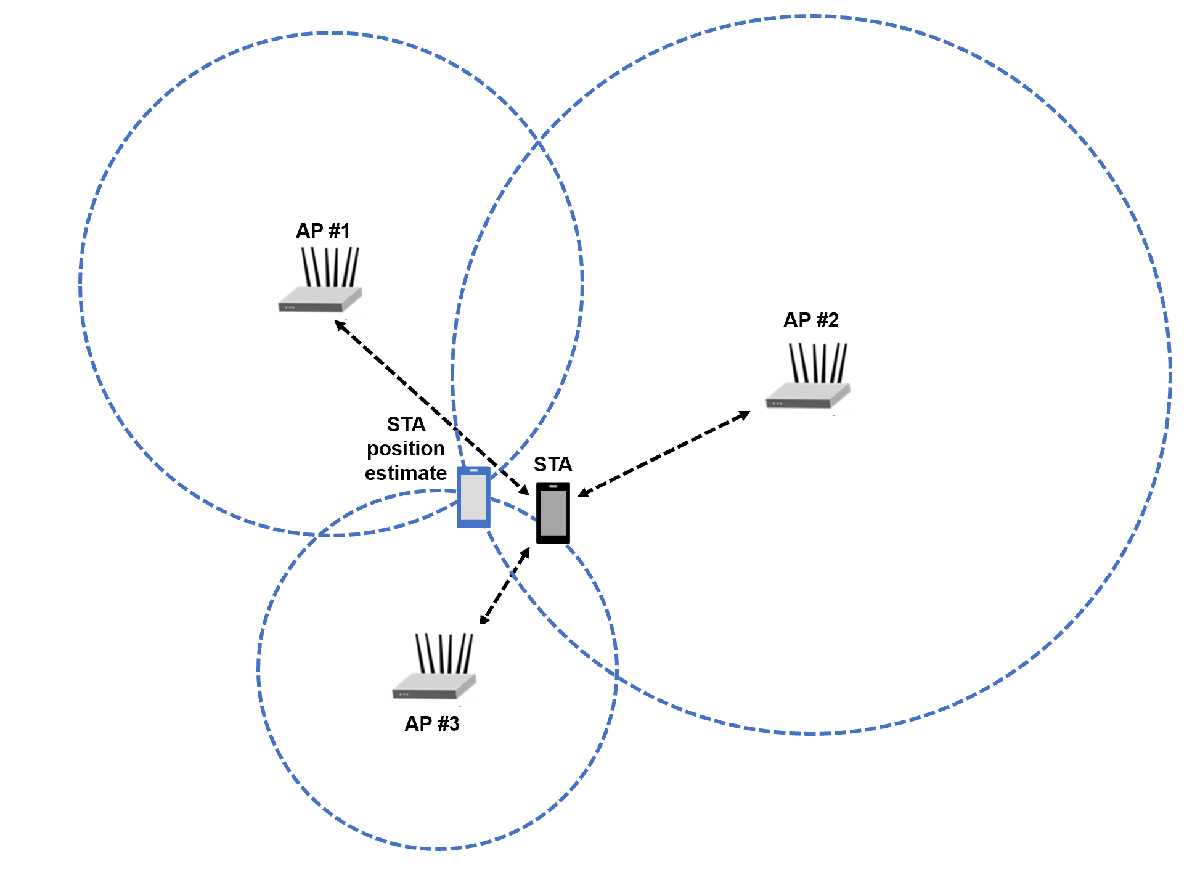Packet Transmission and Reception

This example models the measurement exchange between the STA and APs by performing these steps.

1. Generate a ranging NDP

2. Delay the NDP according to a randomly generated distance between the STA and AP, adding fractional and integer sample delay

3. Pass the waveform through an indoor TGax channel. The example models different channel realizations for different packets

4. Add additive white Gaussian noise (AWGN) to the received waveform. The example uses the same SNR value for all the links between STA and APs

5. Perform synchronization and frequency correction on the received waveform

6. Demodulate the HE-LTF

7. Estimate the channel frequency response from the HE-LTF

8. Estimate the distance by using the MUSIC super-resolution algorithm

9. Combine distance estimates from other STA-AP pairs and trilaterate the position of the STA

This figure illustrates the processing for each STA-AP link.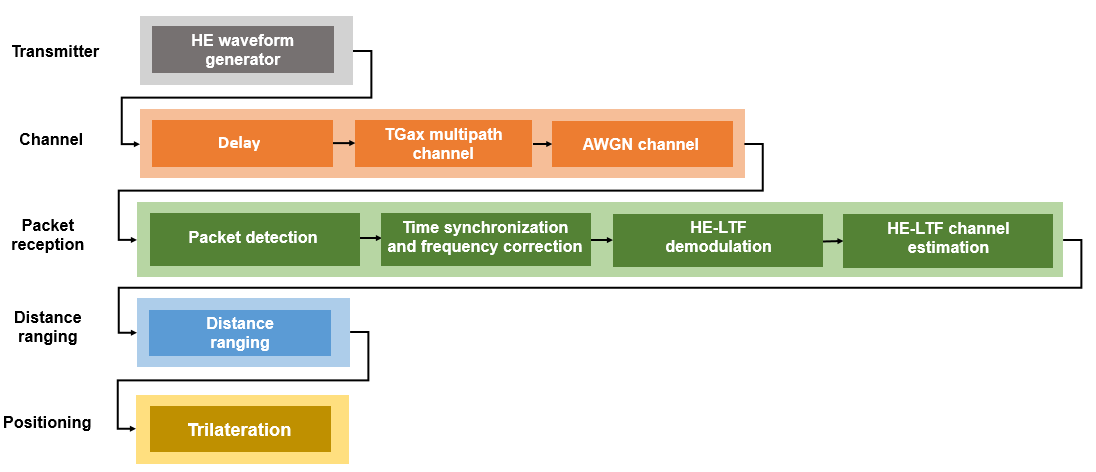Distance Ranging

The example performs a distance ranging measurement between the STA and each AP by capturing the timestamps of the NDP. The STA records the time ${\mathit{t}}_{1}$ (UL ToD) at which it transmits the uplink NDP (UL NDP). The AP then captures the time ${\mathit{t}}_{2}$ (UL ToA) at which it receives the UL NDP and records the time ${\mathit{t}}_{3}$ (DL ToD) at which it transmits the downlink NDP (DL NDP). The STA then captures the time ${\mathit{t}}_{4}$ (DL ToA) at which it receives the DL NDP. This diagram illustrates the measurement sounding phase between a STA and a single AP .The example estimates ${T}_{RTT}$ the round trip time (RTT), by combining these timestamps.

${T}_{RTT}=\left({t}_{4}-{t}_{1}\right)-\left({t}_{3}-{t}_{2}\right)$

The example then computes the distance $\mathit{d}$ between the STA and AP by using this equation.

$d=\frac{{T}_{RTT}}{2}c$ , where $\mathit{c}$ is speed of light

The example estimates ${\mathit{t}}_{2}$ and ${\mathit{t}}_{4}$ by using MUSIC super-resolution. To compute these estimates, the example performs these steps .

1. Interpolate across missing subcarriers from the channel frequency response (CFR), assuming uniform subcarrier spacing

2. Estimate the CFR correlation matrix

3. Decorrelate the multipaths by using spatial smoothing

4. Improve the correlation matrix estimate by performing forward-backward averaging. The example assumes that CFR estimates from multiple spatial streams are different CFR snapshots, and uses all the snapshots for correlation matrix estimation.

5. Run the MUSIC algorithm. Perform eigendecomposition on the correlation matrix to separate it into signal and noise subspaces. Estimate the time-domain delay profile by finding all instances where signal and noise subspaces are orthogonal. The example assumes that the precise signal subspace dimension, equal to the number of multipaths, is known.

6. Determine the ToA by finding the first peak of the recovered multipaths in the estimated delay profile, assumed to be the direct-line-of-sight (DLOS) path

This diagram illustrates the distance ranging process.This plot compares the true multipath delay profile and the MUSIC estimated delay profile for a single 802.11az link simulation.

heRangingPlotDelayProfile()Simulation Parameters

This example performs a ranging and positioning simulation for multiple iterations and SNR points. At each iteration, the AP and the STA exchange multiple uplink and downlink packets. The example estimates the ranging error between the AP and the STA for each iteration by comparing the estimated distance between the AP and the STA with the known distance.

Specify the number of iterations, SNR points, and APs in the networks. To estimate the position of a STA, the network requires a minimum of three APs. For each iteration, use a different random set of AP positions, a different channel realization, and a different AWGN profile. The example generates a cumulative distribution function (CDF) for the absolute ranging error by using the ranging measurements from all iterations and all STA-AP pairs.

numIterations =50; % Number of iterations
snrRange =15:10:35; % SNR points, in dB
numAPs =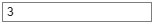3; % Number of APs

802.11az Waveform Configuration

Configure waveform generators for each AP and the STA.

chanBW ='CBW20'; % Channel bandwidth
numTx  =2; % Number of transmit antennas
numRx  =2; % Number of receive antennas
numSTS =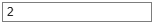2; % Number of space-time streams
numLTFRepetitions =3; % Number of HE-LTF repetitions

Configure the HE ranging NDP parameters of the STA.

cfgSTABase = heRangingConfig;
cfgSTABase.ChannelBandwidth = chanBW;
cfgSTABase.NumTransmitAntennas = numTx;
cfgSTABase.SecureHELTF = true;
cfgSTABase.User{1}.NumSpaceTimeStreams = numSTS;
cfgSTABase.User{1}.NumHELTFRepetition = numLTFRepetitions;
cfgSTABase.GuardInterval = 1.6;

Configure the HE ranging NDP parameters of the APs.

cfgAPBase = cell(1,numAPs);
for iAP = 1:numAPs
cfgAPBase{iAP} = heRangingConfig;
cfgAPBase{iAP}.ChannelBandwidth = chanBW;
cfgAPBase{iAP}.NumTransmitAntennas = numTx;
cfgAPBase{iAP}.SecureHELTF = true;
cfgAPBase{iAP}.User{1}.NumSpaceTimeStreams = numSTS;
cfgAPBase{iAP}.User{1}.NumHELTFRepetition = numLTFRepetitions;
cfgAPBase{iAP}.GuardInterval = 1.6;
end

ofdmInfo = wlanHEOFDMInfo('HE-LTF',chanBW,cfgSTABase.GuardInterval);
sampleRate = wlanSampleRate(chanBW);

Channel Configuration

Configure the WLAN TGax multipath channel by using the wlanTGaxChannel System object™. This System object can generate a channel with a dominant direct path, in which the DLOS path is the strongest path, or a channel with a non-dominant direct path, for which the DLOS path is present, but not the strongest path.

delayProfile ='Model-B'; % TGax channel multipath delay profile

carrierFrequency = 5e9; % Carrier frequency, in Hz
speedOfLight = physconst('lightspeed');

chanBase = wlanTGaxChannel;
chanBase.DelayProfile = delayProfile;
chanBase.NumTransmitAntennas = numTx;
chanBase.SampleRate = sampleRate;
chanBase.CarrierFrequency = carrierFrequency;
chanBase.ChannelBandwidth = chanBW;
chanBase.PathGainsOutputPort = true;
chanBase.NormalizeChannelOutputs = false;

Get channel filter delay and the number of paths

chBaseInfo = info(chanBase);
chDelay = chBaseInfo.ChannelFilterDelay;
numPaths = size(chBaseInfo.PathDelays,2);

Ranging Measurement

Run a ranging simulation with multiple iterations for all STA-AP pairs. Display the ranging mean absolute error (MAE) and the ranging error CDF for each SNR point.

delayULDL = 16e-6; % Time delay between UL NDP ToA and DL NDP ToD, in seconds

numSNR = numel(snrRange);
distEst = zeros(numAPs,numIterations,numSNR);  % Estimated distance
distance = zeros(numAPs,numIterations,numSNR); % True distance
positionSTA = zeros(2,numIterations,numSNR);   % Two-dimensional position of the STA
positionAP= zeros(2,numAPs,numIterations,numSNR); % Two-dimensional positions of the APs
per = zeros(numSNR,1); % Packet error rate (PER)

%parfor isnr = 1:numSNR % Use 'parfor' to speed up the simulation
for isnr = 1:numSNR

% Use a separate channel and waveform configuration object for each parfor stream
chan = chanBase;
cfgAP = cfgAPBase;
cfgSTA = cfgSTABase;

% Initialize ranging error and total failed packet count variables
rangingError = 0;
failedPackets = 0;

% Set random substream index per iteration to ensure that each
% iteration uses a repeatable set of random numbers
stream = RandStream('combRecursive','Seed',123456);
stream.Substream = isnr;
RandStream.setGlobalStream(stream);

% Define the SNR per active subcarrier to account for noise energy in nulls
snrVal = snrRange(isnr) - 10*log10(ofdmInfo.FFTLength/ofdmInfo.NumTones);

for iter = 1:numIterations

% Gennerate random AP positions
[positionSTA(:,iter,isnr),positionAP(:,:,iter,isnr),distanceAllAPs] = heGeneratePositions(numAPs);
distance(:,iter,isnr) = distanceAllAPs;

% Range-based delay
delay = distance(:,iter,isnr)/speedOfLight;
sampleDelay = delay*sampleRate;

% Loop over the number of APs
for ap = 1:numAPs

% ToD of UL NDP (t1)
todUL = randsrc(1,1,0:1e-9:1e-6);

% Loop for both UL and DL transmission

cfgSTA.UplinkIndication = 1; % For UL
% Generate a random secure HE-LTF sequence for the exchange
cfgSTA.User{1}.SecureHELTFSequence = dec2hex(randsrc(1,10,(0:15)))';
cfg = cfgSTA;
else % AP to STA
% Generate a random secure HE-LTF sequence for the exchange
cfgAP{ap}.User{1}.SecureHELTFSequence = dec2hex(randsrc(1,10,(0:15)))';
cfg = cfgAP{ap}; % For DL
end

% Set different channel for UL and DL, assuming that the channel is not reciprocal
reset(chan)

% Generate HE Ranging NDP transmission
tx = heRangingWaveformGenerator(cfg);

% Introduce time delay (fractional and integer) in the transmit waveform
txDelay = heDelaySignal(tx,sampleDelay(ap));

% Pad signal and pass through multipath channel
txMultipath = chan([txDelay;zeros(50,cfg.NumTransmitAntennas)]);

% Pass waveform through AWGN channel
rx = awgn(txMultipath,snrVal);

% Perform synchronization and channel estimation
[chanEstActiveSC,integerOffset] = heRangingSynchronize(rx,cfg);

% Estimate the transmission time between UL and DL
if ~isempty(chanEstActiveSC) % If packet detection is successful

% Estimate fractional delay with MUSIC super-resolution
fracDelay = heRangingTOAEstimate(chanEstActiveSC,ofdmInfo.ActiveFFTIndices, ...
ofdmInfo.FFTLength,sampleRate,numPaths);

integerOffset = integerOffset - chDelay; % Account for channel filter delay
intDelay = integerOffset/sampleRate; % Estimate integer time delay
txTime(l) = intDelay + fracDelay; % Transmission time

else % If packet detection fails
txTime(l) = NaN;
end

end

if ~any(isnan(txTime)) % If packet detection succeeds

% TOA of UL waveform (t2)
toaUL = todUL + txTime(1);

% Time of departure of DL waveform (t3)
todDL = toaUL + delayULDL;

% TOA DL waveform (t4)

% Compute the RTT

% Estimate the distance between the STA and AP
distEst(ap,iter,isnr) = (rtt/2)*speedOfLight;
% Accumulate error to MAE
rangingError = rangingError + abs(distanceAllAPs(ap) - distEst(ap,iter,isnr));

else % If packet detection fails
distEst(ap,iter,isnr) = NaN;
failedPackets = failedPackets + 1;
end

end
end
mae = rangingError/((numAPs*numIterations) - failedPackets); % MAE for successful packets
per(isnr) = failedPackets/(numAPs*numIterations); % PER
if(per(isnr) > 0.01) % Use only successful packets for ranging and positioning
end
disp(['At SNR = ',num2str(snrRange(isnr)),' dB, ','Ranging mean absolute error = ',num2str(mae), ' meters.'])
end
At SNR = 15 dB, Ranging mean absolute error = 0.57082 meters.
At SNR = 25 dB, Ranging mean absolute error = 0.40224 meters.
At SNR = 35 dB, Ranging mean absolute error = 0.25439 meters.
% Reshape to consider all packets within one SNR point as one dataset
rangingError = reshape(abs(distance - distEst),[numAPs*numIterations,numSNR]);
hePlotErrorCDF(rangingError,snrRange)
xlabel('Absolute ranging error (meters)')
title('Ranging Error CDF')Trilateration

Trilaterate the location of the STA in two dimensions by using the distance estimates, then calculate the positioning root-mean-square error (RMSE) for each iteration by using the STA position estimate. Display the average RMSE and its CDF for each SNR point.

positionSTAEst = zeros(2,numIterations,numSNR);
RMSE = zeros(numIterations,numSNR);
for isnr = 1:numSNR
for i = 1:numIterations
positionSTAEst(:,i,isnr) = hePositionEstimate(squeeze(positionAP(:,:,i,isnr)),squeeze(distEst(:,i,isnr)));
end
% Find the RMSE for each iteration, then take the mean of all RMSEs
RMSE = reshape(sqrt(mean(((positionSTAEst-positionSTA).^2),1)),[numIterations numSNR]);
posEr = mean(RMSE(:,isnr),'all','omitnan');
disp(['At SNR = ',num2str(snrRange(isnr)),' dB, ', 'Average RMS Positioning error = ', num2str(posEr), ' meters.'])
end
At SNR = 15 dB, Average RMS Positioning error = 0.61713 meters.
At SNR = 25 dB, Average RMS Positioning error = 0.40481 meters.
At SNR = 35 dB, Average RMS Positioning error = 0.26968 meters.
hePlotErrorCDF(RMSE,snrRange)
xlabel('RMS positioning error (meters)')
title('Positioning Error CDF')Plot the location estimate and the trilateration circles of the last iteration.

hePlotTrilaterationCircles(positionAP(:,:,numIterations,numSNR),positionSTAEst(:,numIterations,numSNR),distEst(:,numIterations,numSNR),snrRange(numSNR),numIterations);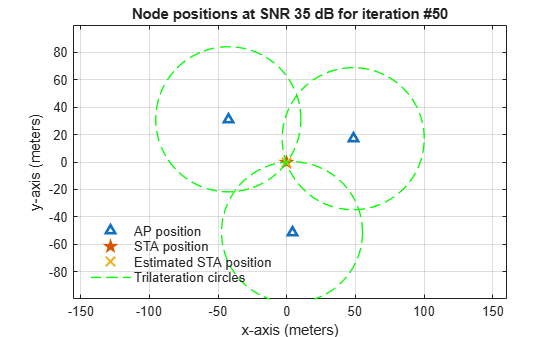Conclusion

This example shows how to use a positioning algorithm with the IEEE® 802.11az™ standard. In particular, the example shows how to estimate the transmit-receive distance between a STA and AP by using MUSIC super-resolution, and how to locate a STA in two dimensions by using the ranging measurements from multiple STA-AP pairs. The example demonstrates the increase in performance of the positioning system at higher SNRs by computing the positioning estimate at multiple SNR points.

Further Exploration

Besides SNR, several important parameters impact positioning performance, such as HE-LTF repetitions, number of spatial streams, higher bandwidths, and channel delay profiles. This figure shows the impact of HE-LTF repetitions on ranging performance. To generate this figure, run a longer simulation with three randomly placed APs and 4000 iterations for a Model-B 2$×$2 MIMO channel of 20 MHz at 20 dB SNR. The figure shows that the ranging error decreases with the increase in HE-LTF repetitions. This decrease occurs because HE-LTF repetitions effectively reduce the noise in the CFR by averaging out multiple CFR estimates.This figure shows the impact of different MIMO configurations on ranging performance. To generate this figure, run a longer simulation with three randomly placed APs and 4000 iterations, generating ranging packets with three HE-LTF repetitions at 20 MHz and specifying a Model-B channel at 20 dB SNR. The figure shows that the ranging error decreases with higher-order MIMO configurations. This decrease occurs because higher-order MIMO configurations produce more CFR snapshots from the different spatial streams available. More CFR snapshots give a better correlation matrix estimate which yields better ToA and distance estimates.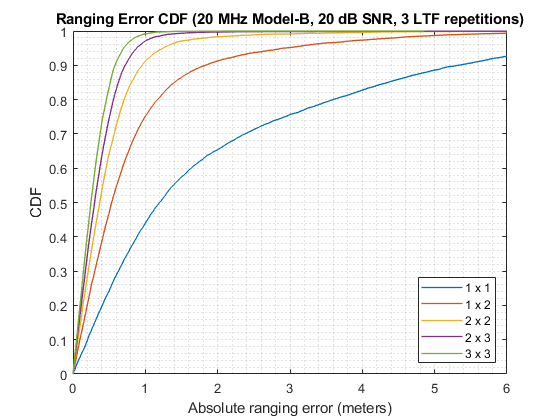References

1. IEEE P802.11az™/D2.0 Draft Standard for Information technology— Telecommunications and information exchange between systems Local and metropolitan area networks— Specific requirements - Amendment 3: Enhancements for positioning.

2. IEEE P802.11ax™/D4.1 Draft Standard for Information technology - Telecommunications and information exchange between systems - Local and metropolitan area networks - Specific requirements - Part 11: Wireless LAN Medium Access Control (MAC) and Physical Layer (PHY) Specifications - Amendment 6: Enhancements for High Efficiency WLAN.

3. Xinrong Li and K. Pahlavan, "Super-resolution TOA estimation with diversity for indoor geolocation," in IEEE Transactions on Wireless Communications, vol. 3, no. 1, pp. 224-234, Jan. 2004, doi: 10.1109/TWC.2003.819035.

WLAN Toolbox DocumentationGet trial now